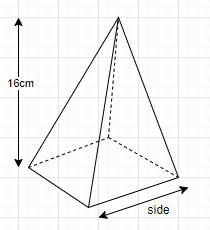QUESTION

# The perimeter of the base of a square pyramid is 96cm and its height is 16cm,a. What is the length of a base edge?b. What is the slant height?c. Find the lateral surface.Perimeter of square base = $4 \times \left( {side} \right)$
$96 = 4 \times side \\ or \\ side = \dfrac{{96}}{4} = 24cm \\$
Let slant height be $l$
$l = \sqrt {{{\left( {\dfrac{{side}}{2}} \right)}^2} + {H^2}} \\ = \sqrt {{{\left( {\dfrac{{24}}{2}} \right)}^2} + {{16}^2}} \\ = \sqrt {{{12}^2} + {{16}^2}} \\ = \sqrt {144 + 256} \\ = \sqrt {400} \\ = 20cm \\$
$= 2 \times side \times l \\ = 2 \times 24 \times 20 \\ = 960c{m^2} \\$